# “The Knowledge Library”

#### Knowledge for All, without Barriers…

##### An Initiative by: Kausik Chakraborty.
09/02/2023 11:56 AM

# “The Knowledge Library”

Knowledge for All, without Barriers…

# “The Knowledge Library”

Knowledge for All, without Barriers……….

# Time and Work Part-2

For Example:

(Q1)Ram can complete a piece of work in 15 days. He worked for 5 days and left. Mohan alone completed the remaining work in 20 days. How long would Mohan take to complete the whole work?

Solution:

Let Ram per day done 1 unit work

Total work done by Ram in 15 days=15 unit

Total work done by Ram in 5 days=5 unit

Remaining work=15-5=10 unit

work done by Mohan in 20 days=10 unit

work done by Mohan in 1 day=10/20=0.5

Time taken by Mohan=15/0.5

=30 days Ans.

(Q2)Ram alone can complete a piece of work in 40 days. He worked for 8 days and left. Sita alone completed the remaining work in 16 days. How long would Ram and Sita together take to complete the work?

Solution:

Let Ram per day done 1 unit work

Total work done by Ram in 40 days=40 unit

Total work done by Ram in 8 days=8 unit

Remaining work=40-8=32 unit

work done by Sita in 16 days=32 unit

work done by Sita in 1 day=32/16

=2 unit per day

Total work done by Ram and Sita in 1 day=(1+2)=3unit per day

Time taken by Ram+Sita=40/3

=13 ¹/3 Ans.

The common and frequently asked a question of Time and work problem with solution and Shortcut Tricks for Bank PO, SSC CGL, SSC CHSL, Railway Exam Type-2.1.

(Q1)A can finish a work in 18 days and B can do the same work in 15 days. B worked for 10 days and left the job. In how many days, A alone can finish the remaining work?

Solution:

Let A= A unit work per day

B= B unit work per day

Now,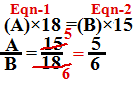[Concept- above equation से हमलोगों ने find out कर लिया कि A एक दिन में 5 unit कम करता है and B एक दिन में 6 unit कम करता है| अब A का value eqn.-1 में रखकर या B का value eqn.-2 में रखकर total work निकाल सकते है ]

Put the value of A in eqn-1, you will get total work

so, total work=5×18=90 unit

Total work done by B in 10 days=10×6=60 unit

Remaining work=90-60

=30 unit

Time taken by A=30/5

=6 days Ans.

Method-2Total work done by B in 10 days=10×6=60 unit

Remaining work=90-60=30 unit

=30 unit

Time taken by A=30/5

=6 days Ans.

The common and frequently asked a question of Time and work problem with solution and Shortcut Tricks for Bank PO, SSC CGL, SSC CHSL, Railway Exam Type-2.2.

(Q1)A can do a certain work in 10 days and B can do same work in 12 days. They started work together but after 5 days A left and B finish the remaining work. In how many days B can complete the remaining work?

Solution:

Let A= A unit work per day

B= B unit work per day

Now,[Concept- above equation से हमलोगों ने find out कर लिया कि A एक दिन में 6 unit कम करता है and B एक दिन में 5 unit कम करता है| अब A का value eqn.-1 में रखकर या B का value eqn.-2 में रखकर total work निकाल सकते है ]

Put the value of A in eqn-1, you will get total work

so, total work=6×10=60 unit

Total work done by A and B in 1 day=(6+5)=11 unit

[Concept: 5 days बाद A चला गया it means A के जाने के पहले दोनों(A and B) 5 days तक एक साथ कम किया होगा तो दोनों मिलकर 5 दिन में कितना कम किया ये हमलोग निकाल लेंगे or फिर उसके बाद total work में से A and B का 5 days का work minus कर देंगे तो इससे Remaining work निकल जाएगा]

work done by A and B in 5 days=11×5=55 unit

Remaining work=60-55

=5 unit

Time taken by B=5/5

=1 day Ans.

Method-2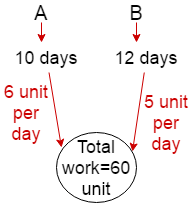Total work done by A and B in 1 day=(6+5)=11 unit

work done by A and B in 5 days=11×5=55 unit

Remaining work=60-55

=5 unit

Time taken by B=5/5

=1 day Ans.

(Q2)A and B can complete a work in 15 days and 10 days respectively. They started doing the work together but after 2 days B had to leave and A alone completed the remaining work. The whole work was completed in:

Solution:

Let A= A unit work per day

B= B unit work per day

Now,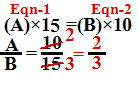[Concept- above equation से हमलोगों ने find out कर लिया कि A एक दिन में 2 unit कम करता है and B एक दिन में 3 unit कम करता है| अब A का value eqn.-1 में रखकर या B का value eqn.-2 में रखकर total work निकाल सकते है ]

Put the value of A in eqn-1, you will get total work

so, total work=2×15=30 unit

Total work done by A and B in 1 day=(2+3)=5 unit

[Concept:2 days बाद B चला गया it means B के जाने के पहले दोनों(A and B) 2 days तक एक साथ कम किया होगा तो दोनों मिलकर 2 दिन में कितना कम किया ये हमलोग निकाल लेंगे or फिर उसके बाद total work में से A and B का 2 days का work minus कर देंगे तो इससे Remaining work निकल जाएगा]

Total work done by A and B in 2 days=5×2=10 unit

Remaining work=30-10

=20 unit

Time taken by A=20/2

=10 days

Hence, total time taken=(2+10)=12days Ans.

Method-2Total work done by A and B in 1 day=(2+3)=5 unit

Total work done by A and B in 2 days=5×2=10 unit

Remaining work=30-10

=20 unit

Time taken by A=20/2

=10 days

Hence, total time taken=(2+10)=12days Ans.

(Q3)A and B can do a piece of work in 45 and 40 days respectively. They began the work together but A left after some days and B finished the remaining work in 23 days. A left after.

Solution:

Let A= A unit work per day

B= B unit work per day

Now,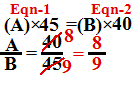[Concept- above equation से हमलोगों ने find out कर लिया कि A एक दिन में 8 unit कम करता है and B एक दिन में 9 unit कम करता है| अब A का value eqn.-1 में रखकर या B का value eqn.-2 में रखकर total work निकाल सकते है ]

Put the value of A in eqn-1, you will get total work

so, total work=8×45=360 unit

[Concept:A कुछ days के बाद चला गया it means A के जाने के बाद सिर्फ B बचा|अब B remaining work को 23 days में complete कर देता है| B एक दिन में 9 unit काम करता है तो 23 दिन में =23×9=207 unit. A के जाने पहले दोनों(A and B) एक साथ कम किया होगा पर कितना दिन तक एक साथ work किया है ये हमलोगों को मालूम नहीं है पर हमलोग ये तो मालूम कर सकते है कि दोनों (A+B)ने मिलकर कितना unit work किया है| so total work done by (A+B)= 360-207=153 unit.]

Total work done by B in 23days=23×9=207 unit

Total Work done by (A+B)=360-207

=153 unit

Total work done by A+B in 1 day=(8+9)=17 unit

Time taken by A+B=153/17

=9 days

hence, A left after 9 days Ans.

Method-2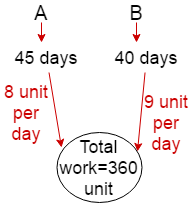Total work done by B in 23days=23×9=207 unit

Total Work done by (A+B)=360-207

=153 unit

Total work done by A+B in 1 day=(8+9)=17 unit

Time taken by(A+B)=153/17

=9 days

hence, A left after 9 days Ans.

(Q4)A can do a piece of work in 20 days while B can finish it in 25 days. B started working and A joined him after 10 days. The whole work is completed in.

Solution:

Let A= A unit work per day

B= B unit work per day

Now,[Concept- above equation से हमलोगों ने find out कर लिया कि A एक दिन में 5 unit कम करता है and B एक दिन में 4 unit कम करता है| अब A का value eqn.-1 में रखकर या B का value eqn.-2 में रखकर total work निकाल सकते है ]

Put the value of A in eqn-1, you will get total work

so, total work=5×20=100 unit

[Concept- Now 10 दिन बाद A ने join किया तो B अकेला 10 days तक work किया है so total work done by B in 10 days =10×4=40 unit. Remaining work=100-40=60 unit, 60 unit work अब A and B दोनों मिलकर करेगा|]

work done by B in 10 days=10×4=40 unit

Remaining work=100-40=60 unit

Total work done by A+B in 1 day=(5+4)=9 unit

Time taken by(A+B)=60/9

=20/3=6 ²/3 days

Total time=10+6 ²/3

=16 ²/3 days Ans.

Method-2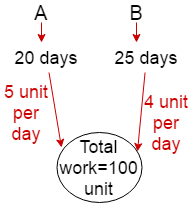work done by B in 10 days=10×4=40 unit

Remaining work=100-40=60 unit

Total work done by A+B in 1 day=(5+4)=9 unit

Time taken by(A+B)=60/9

=20/3=6 ²/3 days

Total time=10+6 ²/3

=16 ²/3 days Ans.

(Q5) A and B can together finish a work in 30 days. They worked at it for 20 days and then B left. The remaining work was done by A alone in 20 more days. A alone can finish the work in.

Solution:

Let work done by (A+B) in per day=1 unit

Total work done by (A+B) in 30 days=30 unit

Total work done by (A+B) in 20 days=20 unit

Now,

Remaining work=30-20=10 unit

work done by A in 20 days=10 unit

∴work done by A in 1 day=10/20 unit

=0.5 unit

Time taken by A=30/0.5

=60 days Ans.

(Q6) A can do a piece of work in 24 days. When he had worked for 4 days. B joined him. If complete work was finished in 16 days, B can alone finish that work in.

Solution:

Let work done by A in per day=1 unit

Total work done by A in 24 days=24 unit

[Concept:जब A 4 days का काम कर चुका था तो B join किया| so work done by A in 4 days=4 unit, then remaining work=24-4=20 unit| 20 unit दोनों(A+B) ने मिलकर किया है| अब question में कह रहा है total work i.e 24 unit work 16 दिन में complete हो रहा है जिसमे 4 days सिर्फ A ने work किया है or remaining 12 days A or B दोनों ने मिलकर किया है |]

work done by A in 4 days=4 unit

remaining work=24-4=20 unit

work done by A+B in 12 days=20 unit

work done by A+B in 1 days=20/12

Now,

A+B=20/12

1+B=20/12

∴ B=2/3

Time taken by B=24/(2/3)

=36 days Ans.

The common and frequently asked a question of Time and work problem with solution and Shortcut Tricks for Bank PO, SSC CGL, SSC CHSL, Railway Exam Type-2.3.

(Q1)A and B alone can complete work in 9 days and 18 days respectively. They worked together; however 3 days before the completion of the work A left. In how many days was the work completed?

Solution:

Let A= A unit work per day

B= B unit work per day

Now,[Concept- above equation से हमलोगों ने find out कर लिया कि A एक दिन में 2 unit कम करता है and B एक दिन में 1 unit कम करता है| अब A का value eqn.-1 में रखकर या B का value eqn.-2 में रखकर total work निकाल सकते है ]

Put the value of A in eqn-1, you will get total work

so, total work=2×9=18 unit

[Concept: A work complete होने के 3 days पहले चला जाता है तो A के चले जाने के बाद सिर्फ B ने 3 days तक work किया है so total work done B in 3 days=3×1=3 unit. total work=2×9=18 unit so remaining work=18-3=15 unit| 15 unit work A and B ने मिलकर किया है]

work done by B in 3 days=3×1=3 unit

Remaining work=18-3=15 unit

Total work done by A+B in 1 day=(2+1)=3 unit

Time taken by(A+B)=15/3

=5 days

Total time=3+5

=8 days Ans.

Method-2work done by B in 3 days=3×1=3 unit

Remaining work=18-3=15 unit

Total work done by A+B in 1 day=(2+1)=3 unit

Time taken by(A+B)=15/3

=5 days

Total time=3+5

=8 days Ans.